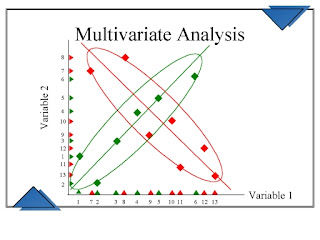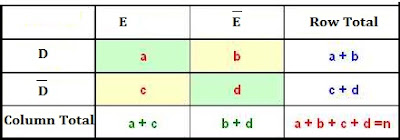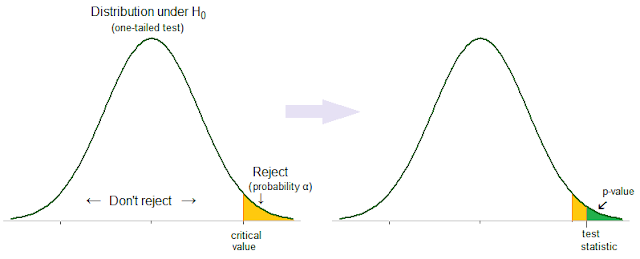## Wednesday, August 9, 2017## Sunday, August 21, 2016We almost always have to deal with limited and varying information. Its very important that how we draw random samples from the population. Let's first learn some key words:

Target Population:
The target population refers to the population to which we would like to apply our estimates and inferences regarding the relationship between disease and exposure.

Study Population:
The study population refers to a convenient subgroup of the population for which appropriate sampling frames are available and we are able to sample.Target population vs study population

Differences between target population and study population introduce selection bias in our results if the study population is not representative of the target population with regard to the disease exposure relationship of concern.
[This does not necessarily require that the study population is representative of all aspects of the target population.]

Points to be considered in choosing the study design:
A good research design should perform the following function:
1. Enable a comparison of variable (such as disease frequency) between two or more groups at one point in time or in some cases, between one group before and after receiving and intervention or being exposed to a risk factor. [Intervention is something like a risk factor]
2.  Allow the comparison to be quantified either in absolute terms (as with risk difference or rate difference) or in relative terms (as with relative risk (RR) or odds ratio (OR).
3.  Permit the investigation to determine when the risk factor and the disease occurred, in order to determine the temporal sequence.
4.  Minimize biases, confounding and other problems that would complicate interpretation of the data.

Types of research design:
1. Observational designs:

1. Population based studies (cross sectional studies)
2. Cohort studies (exposure based sampling
3. Case- control studies (Disease-based sampling)

2. Experimental designs:
1. Randomized control clinical trials (RCCT)
2. Randomized controlled field trials( RCFT)

## Monday, August 8, 2016## What is Relative Risk?

In statistics and epidemiology, the relative risk or risk ratio (RR) is the ratio of probability of an event occurring (as for example, developing a disease) in an exposed group to the probability of the event occurring in a comparison
That is, RR = $\frac{Probability\;of\;event\;under\;exposed}{Probability\;of\;event\;under\;non-exposed}$

Suppose we label the disease outcome by 'D' and the risk factor by 'E' which takes the values 'exposed' or 'not exposed' $\bar E$. Then the relative risk for an outcome D associated with binary risk factor E (i.e. E & $\bar E$ is defined as:
RR = $\frac{P(D।E)}{P(D।\bar E)}$

Consider the table:

RR = $\frac{a/a+c}{b/b+d}$

Description:

$\star$As RR is defined by division of probabilities, RR must be a non-negative number, i.e RR $\ge$ 0.

$\star$ RR =1 implies P(D।E) = P(D।$\bar E)$ i.e D and E are independent.
Note: RR = 1 is a null value being kept in null hypothesis
RR > 1 implies there is a greater risk or probability of D when exposed (E) than when unexposed ($\bar E$).
[i.e P(D।E) > P(D।$\bar E)$
RR <1 implies the reverse i.e the risk is greater among non-exposed.

$\star$ RR provides risk of disease for an exposed individual by multiplying RR by baseline risk.
That is, P(D।E) = RR × baseline risk.

$\star$Its disadvantage is the restricted lower limit i.e RR $\ge$ 0, and implicit upper bound i.e. RR$\le \frac{1}{P(D।\bar E)}$ (always) as P(D।E) cannot be larger than 1.

$\star$ RR is not symmetric i.e. $\frac{P(D।E)}{P(D।\bar E)} \ne \frac{P(D।\bar E)}{P(D।E)}$
Consider an example:
So RR for infant mortality associated with a mother being unmarried at the time of birth is:
$\frac{16712/1213854}{18784/2897205}$ = 2.12
which implies that the risk of an infant death with an unmarried mother is a little more than double the risk when the mother is married.

Another example of Relative Risk:
Suppose, the probability of developing lung cancer among smokers was 20% and among non-smokers was 1%.
RR = $\frac{0.2}{0.01}$ = 20

Interpretation:
Smokers will be 20 times as likely as nonsmokers to develop lung cancer.

Statistical use of RR and its meaning:
RR is used frequently in the statistical analysis of binary outcomes where the outcome of interest has relatively low probability.
It is often suited to clinical trial data where it is used to compare the risk of developing a disease in people not receiving the raw material treatment versus who are recieving an established treatment.

Confidence Interval (CI):
The log of the relative risk has a sampling distribution that is approximately normal with variance that can be estimated by a formula involving the no. of subjects in each group and the event rates in each group (Delta Method). According to this,

CI = log (RR) ± S.E × Z$_\alpha$
where Z$_\alpha$ is the standard score for the chosen level of significance and S.E is the standard error.

## Sunday, August 7, 2016## Test about Population mean (example 1)

In hypothesis testing, we test about a population parameter. In this example, we are testing population mean. Let's see a real life problem followed by its solution.

Problem:
A marketing company has suggested that the cost of monthly cable TV subscription has risen dramatically, which is causing more people to use illegal satellite dishes. Cable TV companies claim that their full cable package subscriptions cost on average $\$$50 a month. The marketing company wants to demonstrate that the cost is significantly greater than \$$50 and randomly selects 40 cable TV subscribers and determines the price they pay for their monthly cable. When the sample of n = 40 subscriptions is randomly selected, the mean of the cable costs is calculated to be$\bar x$= 50.575. Assuming that$\sigma$is known to equal 1.65, test whether the marketing company's claim is true. Solution: Step 1: We set up the hypotheses: Null hypothesis, H$_o$:$\mu$= 50 Alternative hypothesis, H$_1$:$\mu$> 50 Step 2: Desired level of significance We set$\alpha$= 5% or 0.05 i.e. we do not wish to mistake more than 5%. Step 3: Test statistic (TS): TS or Z =$\frac{\bar x - \mu}{\sigma/\sqrt n}$As the sample size is n = 40 ($\gt$30), we may assume, according to Central Limit Theorem (CLT) that TS ~ N(0,1), when null hypothesis is true. Step 4: Value of TS From the problem, we have,$\bar x = \$50.575$
Population mean, $\mu = \$50$Population standard deviation,$\sigma$= 1.65 and sample size, n =40. Hence,$\begin{align}TS, Z = \frac{(50.575-50)}{1.65/\sqrt 40}\\
& = 2.204
\end{align}$Step 5: p-value Since H$_1: \mu>50\begin{align}p-value = P[Z>2.204] \\
& = 1- 0.9875\\
& = 0.0125
\end{align}$Step 6: Decision As p-value$\lt \alpha \;(=0.05)$, we may reject the null hypothesis at 0.05 level of significance. Step 7: Interpretation We have enough evidence to claim that the cost of cable TV subscription has risen. Alternative solution: The above method is called p-value approach. However, we can employ another method called critical region approach. The first three steps are same. Step 4: Construct the rejection region (also called critical region) We assumed$\alpha$= 0.05. Since$H_1 \gt$50, the rejection region is Z$\ge Z_\alpha$. For$\alpha = 0.05, Z_\alpha = 1.645. $Therefore, reject$H_o if Z \ge1.645$. Step 5: Value of Z We have already calculated that, Z = 2.204 Step 6: Decision Since Z$\ge Z_\alpha$, we may reject the null hypothesis ($H_o\$) at 5% level of significance.

Step 7: Interpretation
We have enough evidence to claim that the cost of cable TV subscription has risen.

## Monday, March 21, 2016## Definition and Scope of Epidemilogy

The word epidemiology is derived from the Greek words epi, meaning "upon, among", demos, meaning "people, district", and logos, meaning "study, word, discourse"
Wikipedia: Epidemiology is the study and analysis of the patterns, causes, and effects of health and disease conditions in defined populations. It is the cornerstone of public health, and shapes policy decisions and evidence-based practice by identifying risk factors for disease and targets for preventive healthcare.
Epidemiology as defined by Last is “ the study of the distribution and determinants of health-related states or events in specified populations, and the application of this study to the prevention and control of health problems”
Applications:
Major areas of epidemiological study include disease etiology (the study of causation, or origination), transmission, outbreak investigation, disease surveillance and screening ( in medicine, a strategy used in a population to identify the possible presence of an as-yet-undiagnosed disease in individuals without signs or symptoms ), biomonitoring, and comparisons of treatment effects such as in clinical trials.
Epidemiologists rely on other scientific disciplines like biology to better understand disease processes, statistics to make efficient use of the data and draw appropriate conclusions, social sciences to better understand proximate and distal causes, and engineering for exposure assessment. 
Scope :
A focus of an epidemiological study is the population defined in geographical or other terms; for example, a specific group of hospital patients or factory workers could be the unit of study. A common population used in epidemiology is one selected from a specific area or country at a specific time. This forms the base for defining subgroups with respect to sex, age group or ethnicity. The structures of populations vary between geographical areas and time periods. Epidemiological analyses must take such variation into account. 
Epidemiologists study variations of disease in relation to such factors as age, sex, race, occupational and social characteristics, place of residence, susceptibility, exposure to specific agents, or other pertinent characteristics.
Also of concern are the temporal distribution of disease, examination of trends, cyclical patterns, and intervals between exposure to causative factors and onset of disease. The scope of the field extends from study of the patterns of disease to the causes of disease and to the control or prevention of disease. What distinguishes epidemiology from other clinical sciences is the focus on health problems in population groups rather than in individuals. 
The range of activities that may be at least partly epidemiologic includes determination of the health needs of populations, investigation and control of disease outbreaks, study of environmental and industrial hazards, evaluation of preventive or curative programs or treatments, and evaluation of the effectiveness and efficiency of intervention or control strategies. Many tools of epidemiology are borrowed from other fields such as microbiology, immunology, medicine, statistics, demography, and medical geography.
There is a growing core of purely epidemiologic methodology that includes not only statistical methodology and principles of study design, but a unique way of thinking that is beyond the rote memorization of rules. 
Sources :
 https://yasz82.wordpress.com/2010/08/18/definition-scope-and-uses-of-epidemiology/
 https://en.wikipedia.org/wiki/Epidemiology
 http://www.registrar.ucla.edu/archive/catalog/2005-07/catalog/catalog05-07-3-58.htm

## Monday, February 1, 2016## Saturday, November 21, 2015## Matrix in R programming

#The hash symbols denote comments. They aren't the part of the commands.
#To create the following matrix
# 1  4  7
# 4  7  8
# 6  6  9
matrix(c(1,4,6,4,7,6,7,8,9),3,3)
#By default the values are taken column wise.
#3,3 represents the number of rows and columns
#respectively.
#However only either row or column may be specified.
#In that case, the other will be automatically adjusted.
#so, alternative
matrix(c(1,4,6,4,7,6,7,8,9),3)
#or. If we leave row unspecified, then there needs a comma
#before column number
matrix(c(1,4,6,4,7,6,7,8,9),,3)
#If we want to arrange values row wise
matrix(1:9,,3,byrow=T)
#or in short
matrix(1:9,,3,T)
#By default, it would be
matrix(1:9,3)
#generating 700000 observations from uniform distribution
#in 7 columns
matrix(runif(700000),,7)
#examples: execute to visualize!
matrix(c(1:19,23),,4)
#if there inadequate values, then values would be recycled
#but with a warning.
matrix(c(17,19,20,22,25),3)
#Let's call our initial matrix y and add 1 to all
elements.
y+1
#see [2,3]th element of y where 2 is for row and 3 for
#column
y[2,3]
#all columns of 1st row
y[1,]
#all rows of 2nd column
y[,2]
#Replacing elements e.g, to make all column values of 2nd
#row 10.
y[2,]=10
#Replace 1st three elements of 3rd column by 1, 3 & 4
respectively
y[1:3,3]=c(1,3,4)
#make a matrix having elements 1 in 10 rows and 5 columns
matrix(rep(1,50),10)
#or in short
matrix(1,10,5)
#in the above short code, both row and column number must
#be mentioned.
#A diagonal matrix of order 4
diag(4)
#or of order 5
diag(5)
#Diagnal elements specified
diag(1:5)
#Matrix with diagonal elements 1,3 & 6
diag(c(1,3,6))
#Suppose x is a matrix. to make vector off its diagonal #elements
x=matrix(9:17,3)
diag(x)
#to make a new matrix using diagonal elements of another #matrix, just give above command a name, say, y.
y=diag(x)
#To make a diagonal matrix using diagonal elements of #another matrix.
z=diag(diag(x))

## Sunday, November 8, 2015## The reasons for economies and diseconomies of scale

The economies of scale are the cost saving factors that arise due to the increase in the scale of production. It causes decrease in LAC. It is classified under:
A. Internal or Real economies
B. External economies
A. Internal economies:
i) Economies in production:
Production economies are of two kinds:
a. technical economies:
A large scale expanding firm can afford technically advanced plant and enjoy technical economies. Technical economies include the economies that arise due to the advantage of i) opportunity for using specialized machinery, ii) once-for-all cost of large scale set up and iii) scope of building reserve capacity etc.
b. Advantages of division of labor and specialization:
When firms scale of production expands, more and more qualified workers are employed. Then it becomes possible of division of labor. It increases efficiency and reduces cost of production.
ii) Economies in buying and selling:
A large firm can buy large scale of raw materials and enjoy discounts. So, cost of production reduces. With the increase of the firm, total production increases but the expenditure on advertising the product does not increase proportionately which reduces cost.
iii) Managerial economies:
In a large size firm, it becomes possible to divide the management into specialized departments. They have the opportunity to use advanced techniques of communication, telephones, computer etc. So, managerial cost increases less than proportionately with the increase in production scale.
iv) economies in transportation:
A large size firm may acquire their own means of transportation and can reduce the cost of transportation.

B. External economies:
Consider an example, growth fishing industry encourages growth of firms that supply fishing nets and boats. Competition among such firms (net, boat fishing firms) reduces cost of inputs (for fishing industry). So, cost of production decreases.

Reasons for increase in long run average cost (LAC) or diseconomies of scale:
Diseconomies of scale are disadvantages that arise due to the expansion of production scale and lead to rise in the cost of production. Like economies, diseconomies may be internal and external.
A. Internal diseconomies:
i) Managerial Inefficiency:
With fast expansion of the production scale, personal contacts and communication between a) owners and managers and b) managers and labor get rapidly reduced. this managerial inefficiency leads to rise in cost of production.
ii) Labor inefficiency:
Overcrowding of labor leads to loss of control on labor productivity. On the other hand, increase in the number of workers encourages labor union activities which means simply the loss of output and hence rise in cost of production.

B. External diseconomies:
When all the firm of the industry are expanding, it causes increasing demand of inputs and input price begin to rise causing a rise in cost of production. on the other hand, the law of diminishing returns to scale comes into force due to excessive use of fixed factors. For example, excessive use of cultivable land turns it into a barren land; pumping out water on a large scale for irrigation causes water level to go down resulting in rise in cost of irrigation. These kinds of diseconomies make the LAC more upward
Hey, we've a very popular astronomy portal in Bangla language. If You know Bangla, You'll like it - sky.bishwo.com